Share

NCERT solutions for Class 8 Mathematics chapter 11 - Mensuration

Mathematics Textbook for Class 8

NCERT Mathematics Class 8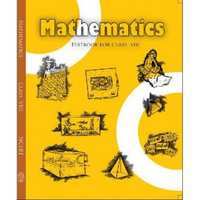Chapter 11: Mensuration

Ex. 11.10Ex. 11.20Ex. 11.30Ex. 11.40

Chapter 11: Mensuration Exercise 11.10 solutions [Page 171]

Ex. 11.10 | Q 1 | Page 171

A square and a rectangular field with measurements as given in the figure have the same perimeter. Which field has a larger area?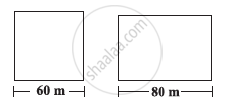Ex. 11.10 | Q 2 | Page 171

Mrs. Kaushik has a square plot with the measurement as shown in the following figure. She wants to construct a house in the middle of the plot. A garden is developed around the house. Find the total cost of developing a garden around the house at the rate of Rs 55 per m2.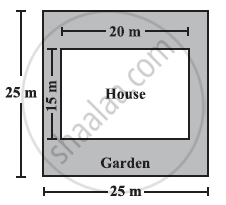Ex. 11.10 | Q 3 | Page 171

The shape of a garden is rectangular in the middle and semi circular at the ends as shown in the diagram. Find the area and the perimeter of the garden [Length of rectangle is 20 − (3.5 + 3.5) metres]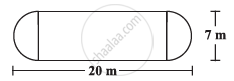Ex. 11.10 | Q 4 | Page 171

A flooring tile has the shape of a parallelogram whose base is 24 cm and the corresponding height is 10 cm. How many such tiles are required to cover a floor of area 1080 m2? (If required you can split the tiles in whatever way you want to fill up the corners).

Ex. 11.10 | Q 5 | Page 171

An ant is moving around a few food pieces of different shapes scattered on the floor. For which food − piece would the ant have to take a longer round? Remember, circumference of a circle can be obtained by using the expression c = 2πr, where r is the radius of the circle.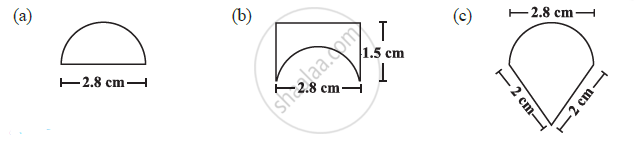Chapter 11: Mensuration Exercise 11.20 solutions [Pages 177 - 178]

Ex. 11.20 | Q 1 | Page 177

The shape of the top surface of a table is a trapezium. Find its area if its parallel sides are 1 m and 1.2 m and perpendicular distance between them is 0.8 m.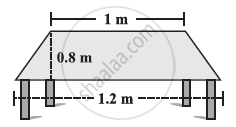Ex. 11.20 | Q 2 | Page 178

The area of a trapezium is 34 cm2 and the length of one of the parallel sides is 10 cm and its height is 4 cm. Find the length of the other parallel side.

Ex. 11.20 | Q 3 | Page 178

Length of the fence of a trapezium shaped field ABCD is 120 m. If BC = 48 m, CD = 17 m and AD = 40 m, find the area of this field. Side AB is perpendicular to the parallel sides AD and BC.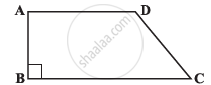Ex. 11.20 | Q 4 | Page 178

The diagonal of a quadrilateral shaped field is 24 m and the perpendiculars dropped on it from the remaining opposite vertices are 8 m and 13 m. Find the area of the field.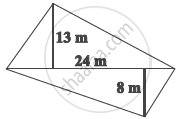Ex. 11.20 | Q 5 | Page 178

The diagonals of a rhombus are 7.5 cm and 12 cm. Find its area.

Ex. 11.20 | Q 6 | Page 178

Find the area of a rhombus whose side is 5 cm and whose altitude is 4.8 cm. If one of its diagonals is 8 cm long, find the length of the other diagonal

Ex. 11.20 | Q 7 | Page 178

The floor of a building consists of 3000 tiles which are rhombus shaped and each of its diagonals are 45 cm and 30 cm in length. Find the total cost of polishing the floor, if the cost per m2 is Rs 4.

Ex. 11.20 | Q 8 | Page 178

Mohan wants to buy a trapezium shaped field. Its side along the river is parallel to and twice the side along the road. It the area of this field is 10500 m2 and the perpendicular distance between the two parallel sides is 100 m, find the length of the side along the river.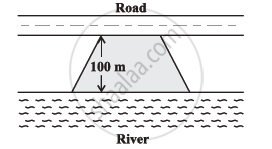Ex. 11.20 | Q 9 | Page 178

Top surface of a raised platform is in the shape of a regular octagon as shown in the figure. Find the area of the octagonal surface.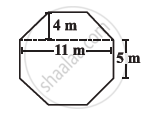Ex. 11.20 | Q 10 | Page 178

There is a pentagonal shaped park as shown in the figure.

For finding its area Jyoti and Kavita divided it in two different ways.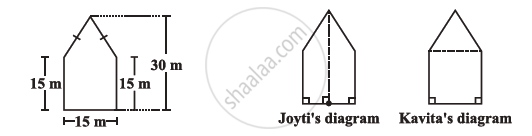Find the area of this park using both ways. Can you suggest some other way of finding its area?

Ex. 11.20 | Q 11 | Page 178

Diagram of the adjacent picture frame has outer dimensions = 24 cm × 28 cm and inner dimensions 16 cm × 20 cm. Find the area of each section of the frame, if the width of each section is same.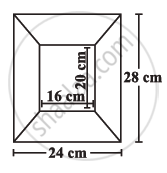Chapter 11: Mensuration Exercise 11.30 solutions [Page 186]

Ex. 11.30 | Q 1 | Page 186

There are two cuboidal boxes as shown in the adjoining figure. Which box requires the lesser amount of material to make?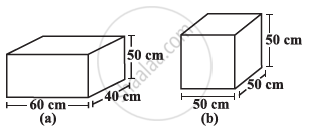Ex. 11.30 | Q 2 | Page 186

A suitcase with measures 80 cm × 48 cm × 24 cm is to be covered with a tarpaulin cloth. How many metres of tarpaulin of width 96 cm is required to cover 100 such suitcases?

Ex. 11.30 | Q 3 | Page 186

Find the side of a cube whose surface area is 600 cm2.

Ex. 11.30 | Q 4 | Page 186

Rukhsar painted the outside of the cabinet of measure 1 m × 2 m × 1.5 m. How much surface area did she cover if she painted all except the bottom of the cabinet?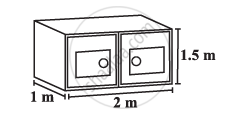Ex. 11.30 | Q 5 | Page 186

Daniel is painting the walls and ceiling of a cuboidal hall with length, breadth and height of 15 m, 10 m and 7 m respectively. From each can of paint 100 m2 of area is painted. How many cans of paint will she need to paint the room?

Ex. 11.30 | Q 6 | Page 186

Describe how the two figures at the right are alike and how they are different. Which box has larger lateral surface area?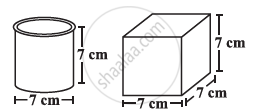Ex. 11.30 | Q 7 | Page 186

A closed cylindrical tank of radius 7 m and height 3 m is made from a sheet of metal. How much sheet of metal is required?

Ex. 11.30 | Q 8 | Page 186

The lateral surface area of a hollow cylinder is 4224 cm2. It is cut along its height and formed a rectangular sheet of width 33 cm. Find the perimeter of rectangular sheet?

Ex. 11.30 | Q 9 | Page 186

A road roller takes 750 complete revolutions to move once over to level a road. Find the area of the road if the diameter of a road roller is 84 cm and length is 1 m.

Ex. 11.30 | Q 10 | Page 186

A company packages its milk powder in cylindrical container whose base has a diameter of 14 cm and height 20 cm. Company places a label around the surface of the container (as shown in the figure). If the label is placed 2 cm from top and bottom, what is the area of the label.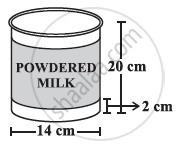Chapter 11: Mensuration Exercise 11.40 solutions [Pages 191 - 192]

Ex. 11.40 | Q 1 | Page 191

Given a cylindrical tank, in which situation will you find surface area and in which situation volume.

(a) To find how much it can hold

(b) Number of cement bags required to plaster it

(c) To find the number of smaller tanks that can be filled with water from it.

Ex. 11.40 | Q 2 | Page 191

Diameter of cylinder A is 7 cm, and the height is 14 cm. Diameter of cylinder B is 14 cm and height is 7 cm. Without doing any calculations can you suggest whose volume is greater? Verify it by finding the volume of both the cylinders. Check whether the cylinder with greater volume also has greater surface area?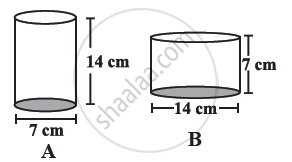Ex. 11.40 | Q 3 | Page 191

Find the height of a cuboid whose base area is 180 cm2 and volume is 900 cm3?

Ex. 11.40 | Q 4 | Page 191

A cuboid is of dimensions 60 cm × 54 cm × 30 cm. How many small cubes with side 6 cm can be placed in the given cuboid?

Ex. 11.40 | Q 5 | Page 191

Find the height of the cylinder whose volume is 1.54 m3 and diameter of the base is 140 cm?

Ex. 11.40 | Q 6 | Page 191

A milk tank is in the form of cylinder whose radius is 1.5 m and length is 7 m. Find the quantity of milk in litres that can be stored in the tank?

Ex. 11.40 | Q 7.1 | Page 191

If each edge of a cube is doubled how many times will its surface area increase?

Ex. 11.40 | Q 7.2 | Page 191

If each edge of a cube is doubled, how many times will its volume increase?

Ex. 11.40 | Q 8 | Page 192

Water is pouring into a cubiodal reservoir at the rate of 60 litres per minute. If the volume of reservoir is 108 m3, find the number of hours it will take to fill the reservoir.

Chapter 11: Mensuration

Ex. 11.10Ex. 11.20Ex. 11.30Ex. 11.40

NCERT Mathematics Class 8NCERT solutions for Class 8 Mathematics chapter 11 - Mensuration

NCERT solutions for Class 8 Maths chapter 11 (Mensuration) include all questions with solution and detail explanation. This will clear students doubts about any question and improve application skills while preparing for board exams. The detailed, step-by-step solutions will help you understand the concepts better and clear your confusions, if any. Shaalaa.com has the CBSE Mathematics Textbook for Class 8 solutions in a manner that help students grasp basic concepts better and faster.

Further, we at Shaalaa.com are providing such solutions so that students can prepare for written exams. NCERT textbook solutions can be a core help for self-study and acts as a perfect self-help guidance for students.

Concepts covered in Class 8 Mathematics chapter 11 Mensuration are Area of Trapezium, Area of a General Quadrilateral, Area of a Polygon, Concept of Solid Shapes, Concept of Cuboid, Concept of Cube, Concept of Cylinders, Conept of Cylinder, Volume and Capacity, Introduction of Mensuration, Concept of Cuboid, Concept of Cube.

Using NCERT Class 8 solutions Mensuration exercise by students are an easy way to prepare for the exams, as they involve solutions arranged chapter-wise also page wise. The questions involved in NCERT Solutions are important questions that can be asked in the final exam. Maximum students of CBSE Class 8 prefer NCERT Textbook Solutions to score more in exam.

Get the free view of chapter 11 Mensuration Class 8 extra questions for Maths and can use Shaalaa.com to keep it handy for your exam preparation

S